# Surface Area of a Triangular Prism Online Quiz

#### Surface Areas and Volumes

117 Lectures 6.5 hours

#### Class 10th - Surface Areas and Volumes

20 Lectures 1 hours

#### Class 10th - Surface Areas and Volumes (Hindi)

10 Lectures 47 mins

Following quiz provides Multiple Choice Questions (MCQs) related to Surface Area of a Triangular Prism. You will have to read all the given answers and click over the correct answer. If you are not sure about the answer then you can check the answer using Show Answer button. You can use Next Quiz button to check new set of questions in the quiz.Q 1 - Find the surface area of the following triangular prism.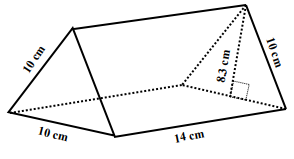### Explanation

Step 1:

Area of triangle base A = 10×10 × $\frac{1.73}{4}$ = 43.25 sq cm; height of prism h = 14 cm

Step 2:

Surface area of triangular prism = 2A + (a+b+c)h

= 2 × 43.25 + 30 × 14

= 506.5 square cm

Q 2 - Find the surface area of the following triangular prism.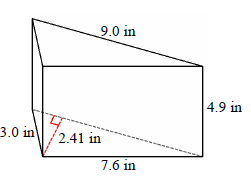### Explanation

Step 1:

Area of triangle base A = $\sqrt{s\left ( s-a \right )\left ( s-b \right )\left ( s-c \right )}$

= $\sqrt{9.8\left ( 9.8-3 \right )\left ( 9.8 -9\right )\left ( 9.8-7.6 \right ) }$

= 10.83 sq in; height of prism h = 4.9 in

Step 2:

Surface area of triangular prism = 2A + (a+b+c)h

= 2 × 10.83 + (3+9+7.6) × 4.9

= 117.7 square in

Q 3 - Find the surface area of the following triangular prism.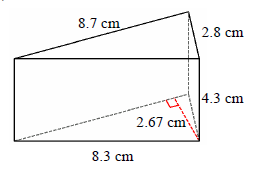### Explanation

Step 1:

Area of triangle base A = 11.62 sq cm; height of prism h = 4.3 cm

Step 2:

Surface area of triangular prism = 2A + (a+b+c)h

= 2 × 11.62 + (8.3+8.7+2.8) × 4.3

= 108.37 square cm

Q 4 - Find the surface area of the following triangular prism.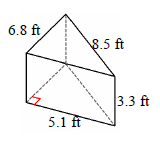### Explanation

Step 1:

Area of triangle base A = 17.34 sq ft; height of prism h = 3.3 ft

Step 2:

Surface area of triangular prism = 2A + (a+b+c)h

= 2 × 17.34 + (6.8+8.5+5.1) × 3.3

= 102 square ft

Q 5 - Find the surface area of the following triangular prism.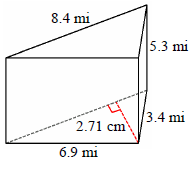### Explanation

Step 1:

Area of triangle base A = 11.38 sq mi; height of prism h = 5.3 mi

Step 2:

Surface area of triangular prism = 2A + (a+b+c)h

= 2 × 11.38 + (6.9+3.4+8.4) × 5.3

= 121.87 square mi

Q 6 - Find the surface area of the following triangular prism.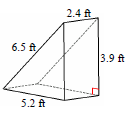### Explanation

Step 1:

Area of triangle base A = 10.14 sq ft; height of prism h = 2.4 ft

Step 2:

Surface area of triangular prism = 2A + (a+b+c)

= 2 × 10.14 + (5.2+3.9+6.5) × 2.4

= 57.72 square ft

Q 7 - Find the surface area of the following triangular prism.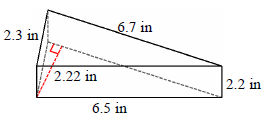### Explanation

Step 1:

Area of triangle base A = 7.45 sq in; height of prism h = 2.2 in

Step 2:

Surface area of triangular prism = 2A + (a+b+c)h

= 2 × 7.45 + (2.3+6.5+6.7) × 2.2

= 48.99 square in

Q 8 - Find the surface area of the following triangular prism.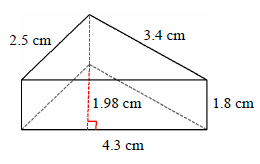### Explanation

Step 1:

Area of triangle base A = 4.25 sq cm; height of prism h = 1.8 cm

Step 2:

Surface area of triangular prism = 2A + (a+b+c)h

= 2 × 4.25 + (2.5 + 3.4 + 4.3) × 1.8

= 26.85 square cm

Q 9 - Find the surface area of the following triangular prism.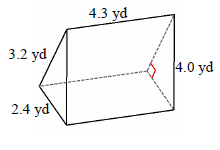### Explanation

Step 1:

Area of triangle base A = 3.84 sq yd; height of prism h = 4.3 yd

Step 2:

Surface area of triangular prism = 2A + (a+b+c)h

= 2 × 3.84 + (2.4 + 3.2 + 4) × 4.3

= 48.96 square yd

Q 10 - Find the surface area of the following triangular prism.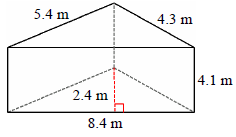### Explanation

Step 1:

Area of triangle base A = 10.1sq m ; height of prism h = 4.1 m

Step 2:

Surface area of triangular prism = 2A + (a+b+c)h

= 2 × 10.1 + (4.3 + 5.4 + 8.4) × 4.1

= 94.41 square m

surface_area_of_a_triangular_prism.htm